Pit optimization has been widely used in the minerals industry for many decades.  However, for bedded deposits there is an inherent problem with the existing technique.  This paper will firstly describe the methodology in current use, then present the inherent problem with this method, and finally explain the solution to the problem.

Current Methodology

Optimization is based on building a pattern of 3D blocks which are then valued.  Values and costs are assigned to each individual block as shown in Figure 1.  Ore is assigned a value calculated on quality/grade, mining cost, haulage cost, processing cost and recovery.  Waste is assigned a mining cost and haulage cost.  The sum of the values in a block is the total value of that block.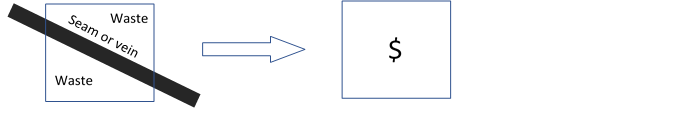Figure 1 – Assignment of value to each block

Figure 2 shows a vertical cross-section through an example deposit with a seam or vein dipping to the right.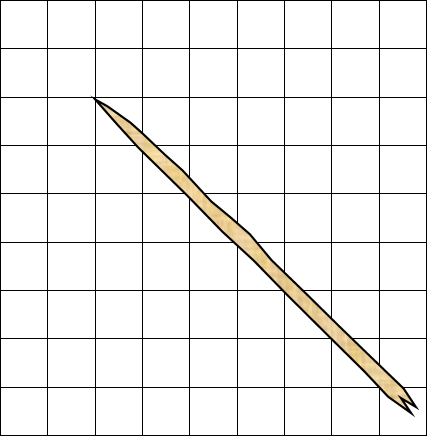Figure 2 – Vertical cross-section showing seam or vein

Figure 3 shows the vertical cross-section after the assignment of values to each block. In this figure the costs of mining waste is taken as \$4.  The ore blocks will have a net positive value.  The first row consists of what is termed “air blocks”.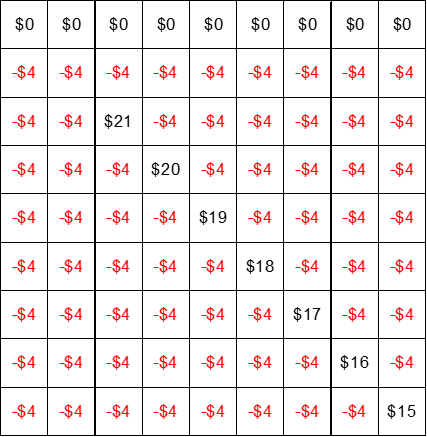Figure 3 – Vertical cross-section showing assignment of values

The next step in pit optimization is to sum the blocks in each column vertically.  This results in the values presented in Figure 4.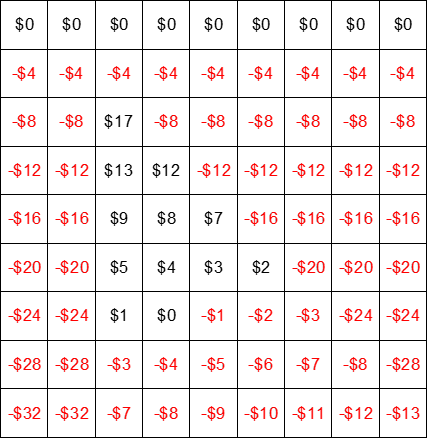Figure 4 – Blocks after vertical summation

In an open cut mine, mining can progress in one of three directions as shown in Figure 5.  In this case it is assumed that the stable wall angle is 45°.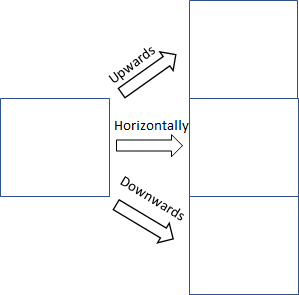Figure 5 – Mining directions

Starting in the top left corner the next step is to sum the blocks along the mining directions shown in Figure 5 in the direction which will give the maximum cumulative value and mark the direction from which the summation was made.  This results in the values presented in Figure 6.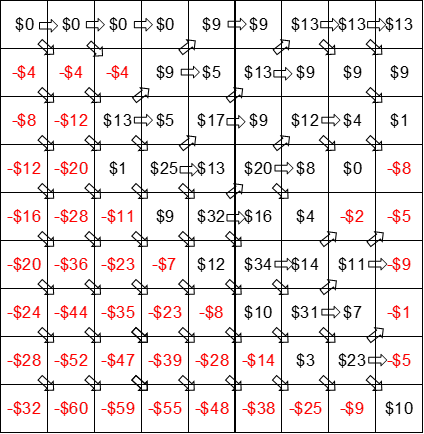Figure 6 – Blocks after summing for maximum value & marking source

The next step is to trace the arrows back along the mining directions starting in the top right corner as shown in Figure 7.  The resultant trace is the base of the optimum pit.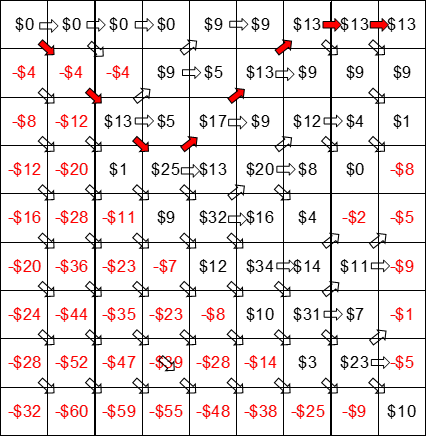Figure 7 – The highlighted trace of the optimum pit floor

The inherent problem for bedded deposits

Because the blocks are an approximation to the floor/footwall  of the vein or seam some material below the seam or vein will be included in the assessment of the optimum pit as shown in Figure 8.  This material is called underburden.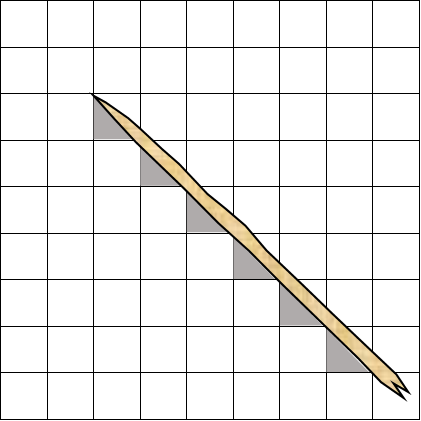Figure 8 – Section highlighting the underburden problem

For any set of costs, including the underburden causes the optimum pit to be pessimistic because, except in cases where the the stable pit wall angle is shallower than the dip of the seam or vein, the underburden is included in the assessment of the mining economics even though it will never be mined.

Creating smaller blocks reduces the problem but does not remove the problem.  This staircase effect is illustrated in the three-dimensional display of part of an optimum pit presented in Figure 9.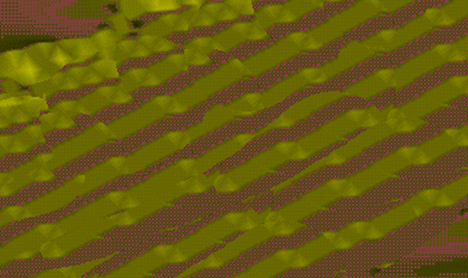Figure 9 – Three-dimensional view highlighting staircase effect

Solution to the problem

It has already been shown that using blocks with fixed vertical dimensions does not adequately model a bedded deposit or flat-dipping vein due to the underburden problem.  This is the reason that bedded deposits are typically modelled using two-dimensional grids rather than three-dimensional blocks.  In two-dimensional grids each XY grid cell contains the actual vertical elevaton of the surface being modelled rather than relying on the approximation offered by three-dimensional blocks.  This means that the upper and lower surfaces of the seam or vein are accurately modelled.  As a result, instead of a block containing a mix of waste and ore each mining block contains only ore or waste.  Noting also that the thinner the seams or veins the greater the problem created by using blocks with fixed vertical dimensions.

Figure 10 compares the optimum pit obtained using a 3D block model with the optimum pit obtained using a 2D gridded model.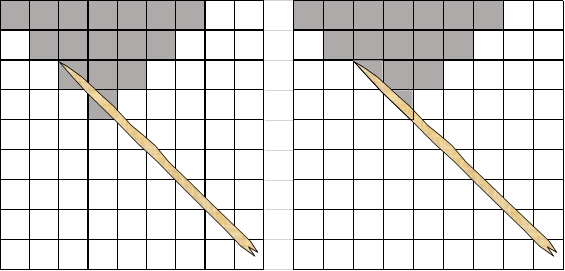Figure 10 – Comparison between optimum pit using 3D block model (left) and 2D grid model (right)

The advantage of using optimization based directly on a 2D grid model for a seam or vein rather than using a 3D block model becomes more pronounced as the dip angle of the seam or vein reduces.  This is highlighted in Figure 11 which shows that, in this instance, the optimum pit created using the 2D grid model optimization technique recovers approximately 25% more ore than the optimum pit based on a 3D block model for the same economic inputs.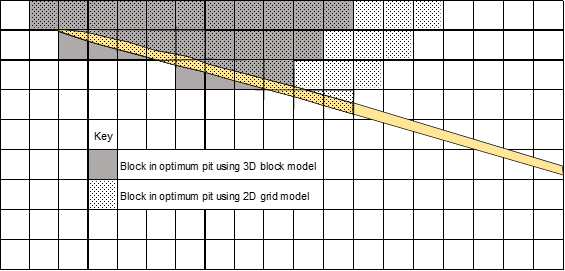Figure 11 – Increased advantage of optimum pit based on 2D grid model with reduction in dip angle

It is clear that applying 2D grid pit optimization to a set of volumes modelled using two-dimensional grids produces an optimum pit shell which follows the bedded structure and gives a superior result to optimization based on three-dimensional blocks.  An example of the output is illustrated in Figure 12 where it can be seen how the pit floor follows the basal bedded structure.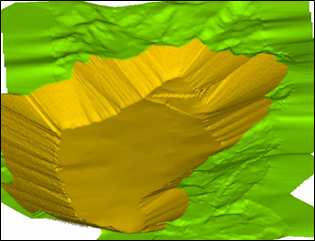Figure 12 – Optimization with pit floor following basal bed structure

This approach has all of the advantages of current commercially available pit optimizers, with the added benefits of:

1. The lower limits of the resultant optimum pit follows the floor, or footwall, of the seam or vein, i.e. there is no underburden, and;
2. The optimization result more accuractely reflects the specified economics.

To the best of our knowledge this technique is available exclusively through ASEAMCO.